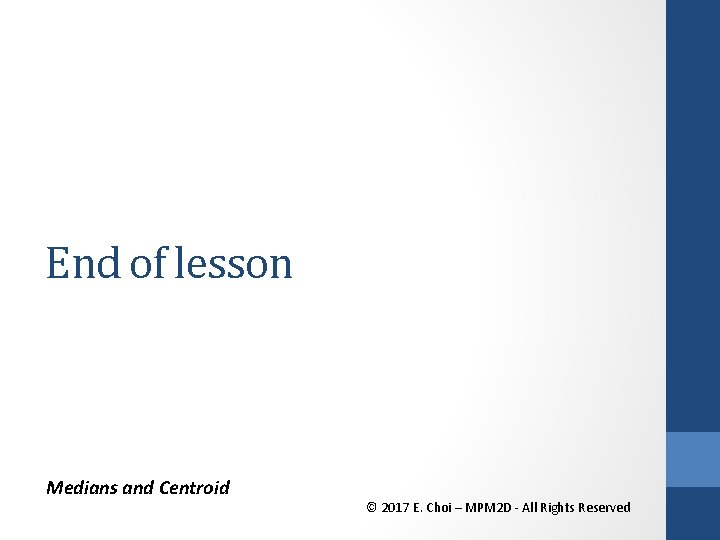• Slides: 15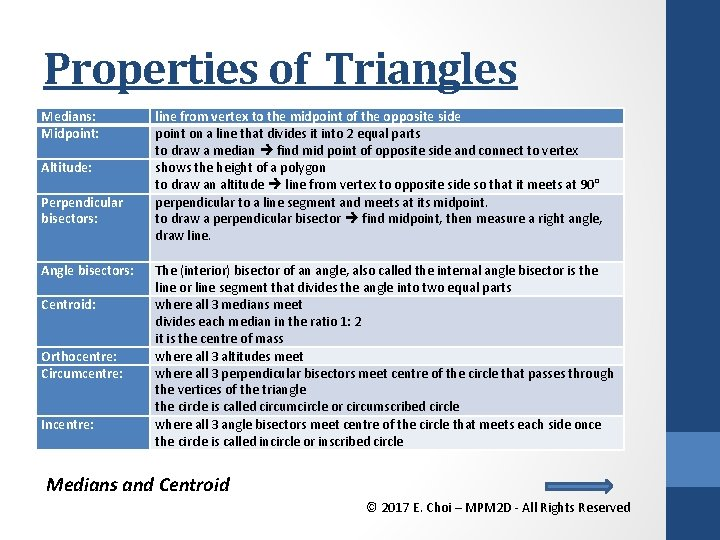Properties of Triangles Medians: Midpoint: Altitude: Perpendicular bisectors: Angle bisectors: Centroid: Orthocentre: Circumcentre: Incentre: line from vertex to the midpoint of the opposite side point on a line that divides it into 2 equal parts to draw a median find mid point of opposite side and connect to vertex shows the height of a polygon to draw an altitude line from vertex to opposite side so that it meets at 90° perpendicular to a line segment and meets at its midpoint. to draw a perpendicular bisector find midpoint, then measure a right angle, draw line. The (interior) bisector of an angle, also called the internal angle bisector is the line or line segment that divides the angle into two equal parts where all 3 medians meet divides each median in the ratio 1: 2 it is the centre of mass where all 3 altitudes meet where all 3 perpendicular bisectors meet centre of the circle that passes through the vertices of the triangle the circle is called circumcircle or circumscribed circle where all 3 angle bisectors meet centre of the circle that meets each side once the circle is called incircle or inscribed circle Medians and Centroid © 2017 E. Choi – MPM 2 D - All Rights Reserved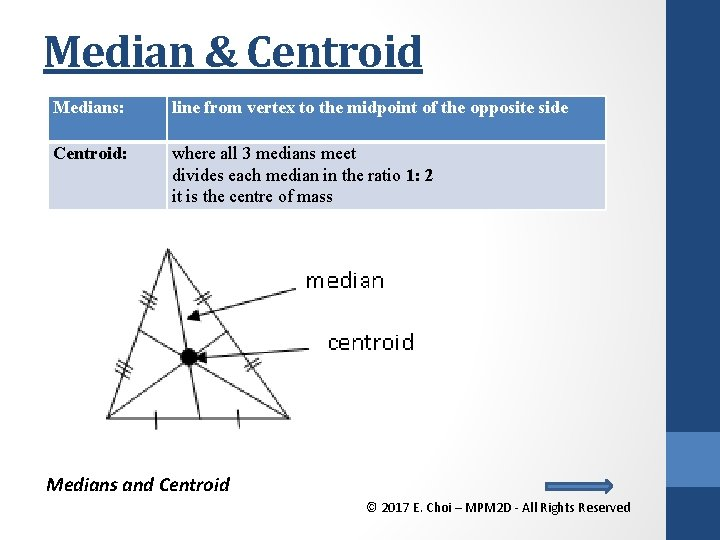Median & Centroid Medians: line from vertex to the midpoint of the opposite side Centroid: where all 3 medians meet divides each median in the ratio 1: 2 it is the centre of mass Medians and Centroid © 2017 E. Choi – MPM 2 D - All Rights Reserved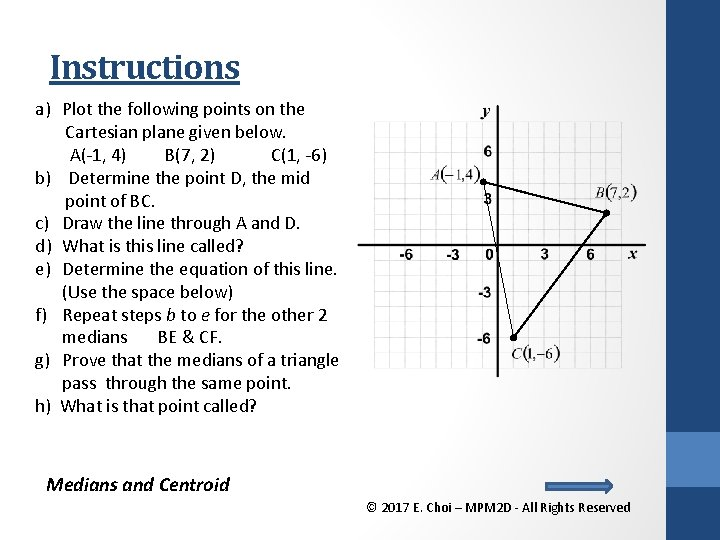Instructions a) Plot the following points on the Cartesian plane given below. A(-1, 4) B(7, 2) C(1, -6) b) Determine the point D, the mid point of BC. c) Draw the line through A and D. d) What is this line called? e) Determine the equation of this line. (Use the space below) f) Repeat steps b to e for the other 2 medians BE & CF. g) Prove that the medians of a triangle pass through the same point. h) What is that point called? Medians and Centroid © 2017 E. Choi – MPM 2 D - All Rights Reserved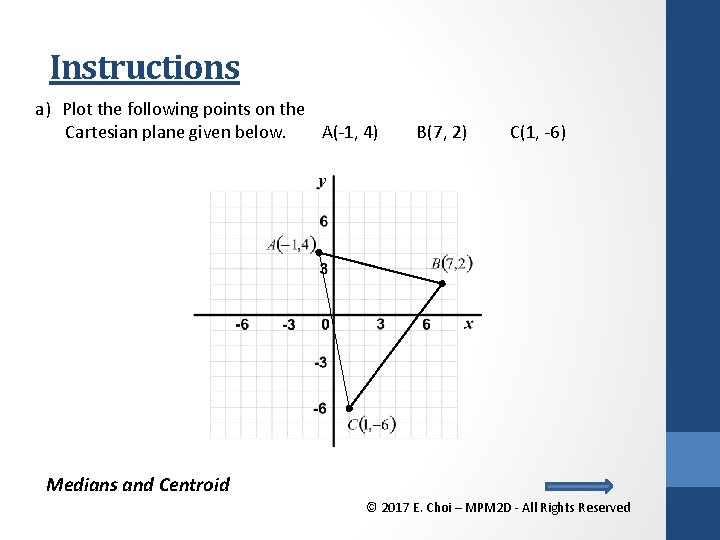Instructions a) Plot the following points on the Cartesian plane given below. A(-1, 4) B(7, 2) C(1, -6) Medians and Centroid © 2017 E. Choi – MPM 2 D - All Rights Reserved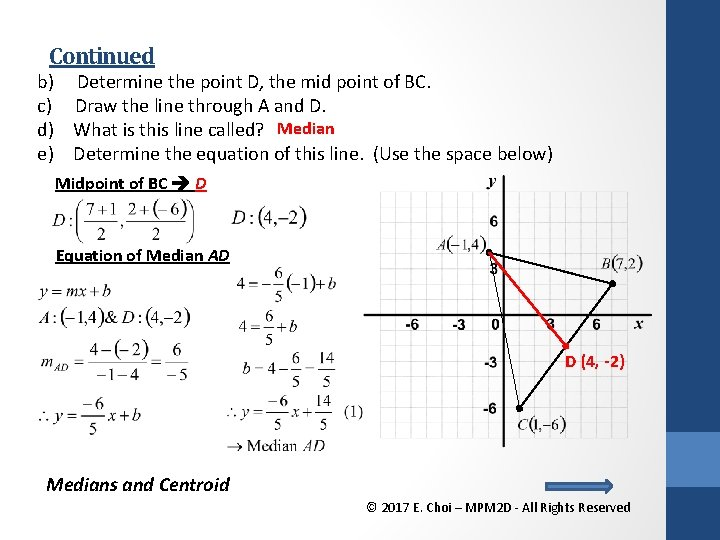Continued b) Determine the point D, the mid point of BC. c) Draw the line through A and D. d) What is this line called? Median e) Determine the equation of this line. (Use the space below) Midpoint of BC D Equation of Median AD D (4, -2) Medians and Centroid © 2017 E. Choi – MPM 2 D - All Rights Reserved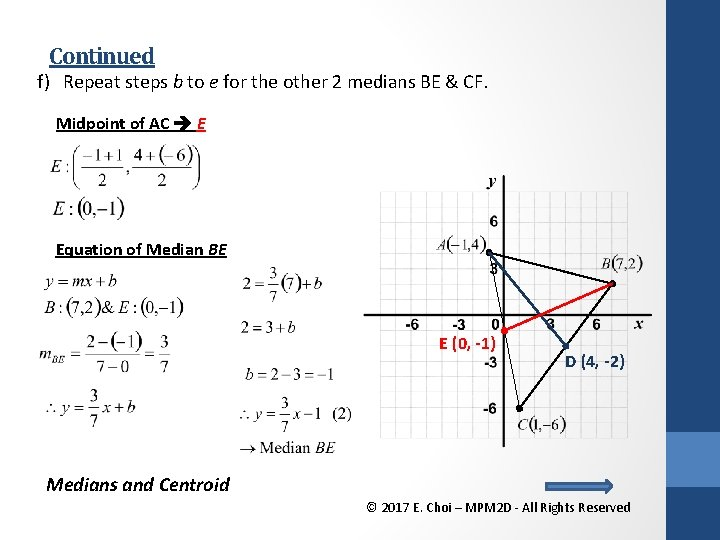Continued f) Repeat steps b to e for the other 2 medians BE & CF. Midpoint of AC E Equation of Median BE E (0, -1) D (4, -2) Medians and Centroid © 2017 E. Choi – MPM 2 D - All Rights Reserved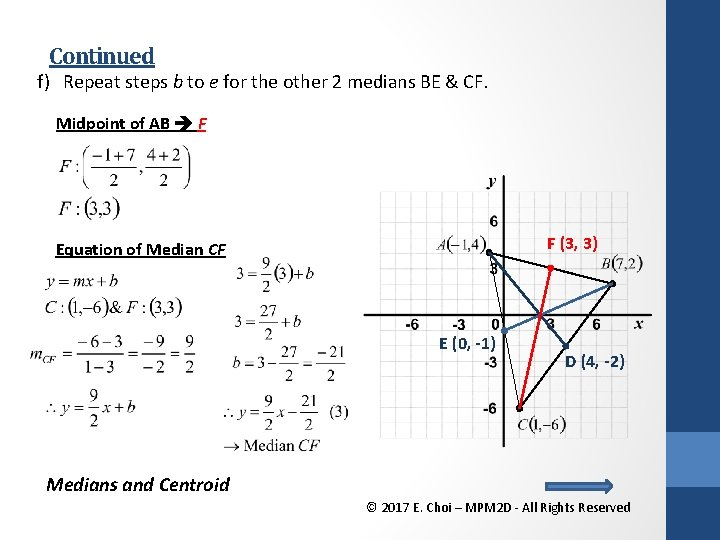Continued f) Repeat steps b to e for the other 2 medians BE & CF. Midpoint of AB F F (3, 3) Equation of Median CF E (0, -1) D (4, -2) Medians and Centroid © 2017 E. Choi – MPM 2 D - All Rights ReservedContinued: Point of Intersection (Centroid) g) Prove that the medians of a triangle pass through the same point. By method of comparison with equation 1 and 2, find the point of intersection and check with all three equations. Verify that all medians intersect at the same point. Recall: Let (1) = (2) F (3, 3) E (0, -1) D (4, -2) Medians and Centroid © 2017 E. Choi – MPM 2 D - All Rights Reserved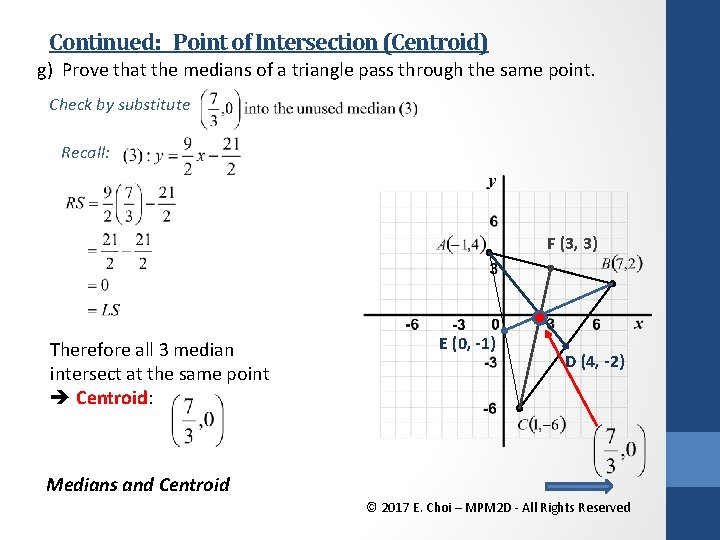Continued: Point of Intersection (Centroid) g) Prove that the medians of a triangle pass through the same point. Check by substitute Recall: F (3, 3) Therefore all 3 median intersect at the same point Centroid: E (0, -1) D (4, -2) Medians and Centroid © 2017 E. Choi – MPM 2 D - All Rights ReservedCentroid Formula!! Let’s redo the same example by introducing the Centroid formula Recall: A(-1, 4) B(7, 2) C(1, -6) F (3, 3) E (0, -1) D (4, -2) Same!!! Medians and Centroid © 2017 E. Choi – MPM 2 D - All Rights ReservedMedian & Centroid Medians: line from vertex to the midpoint of the opposite side Centroid: where all 3 medians meet divides each median in the ratio 1: 2 it is the centre of mass Centroid: The point where three medians of a triangle intersect. Also called the centre of mass or balance point. Medians and Centroid © 2017 E. Choi – MPM 2 D - All Rights Reserved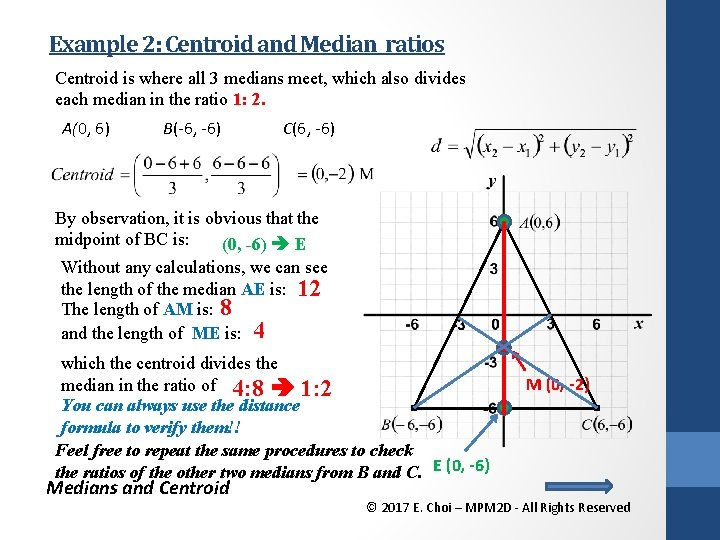Example 2: Centroid and Median ratios Centroid is where all 3 medians meet, which also divides each median in the ratio 1: 2. A(0, 6) B(-6, -6) C(6, -6) By observation, it is obvious that the midpoint of BC is: (0, -6) E Without any calculations, we can see the length of the median AE is: 12 The length of AM is: 8 and the length of ME is: 4 which the centroid divides the median in the ratio of 4: 8 1: 2 You can always use the distance formula to verify them!! Feel free to repeat the same procedures to check the ratios of the other two medians from B and C. E (0, -6) Medians and Centroid M (0, -2) © 2017 E. Choi – MPM 2 D - All Rights ReservedHomework Work sheet: Extra practice #1 a-d Text: • P. 173 #2, #4, 13, 14 • P. 194 #1, 8, 12, 18 Check the website for updates Medians and Centroid © 2017 E. Choi – MPM 2 D - All Rights Reserved